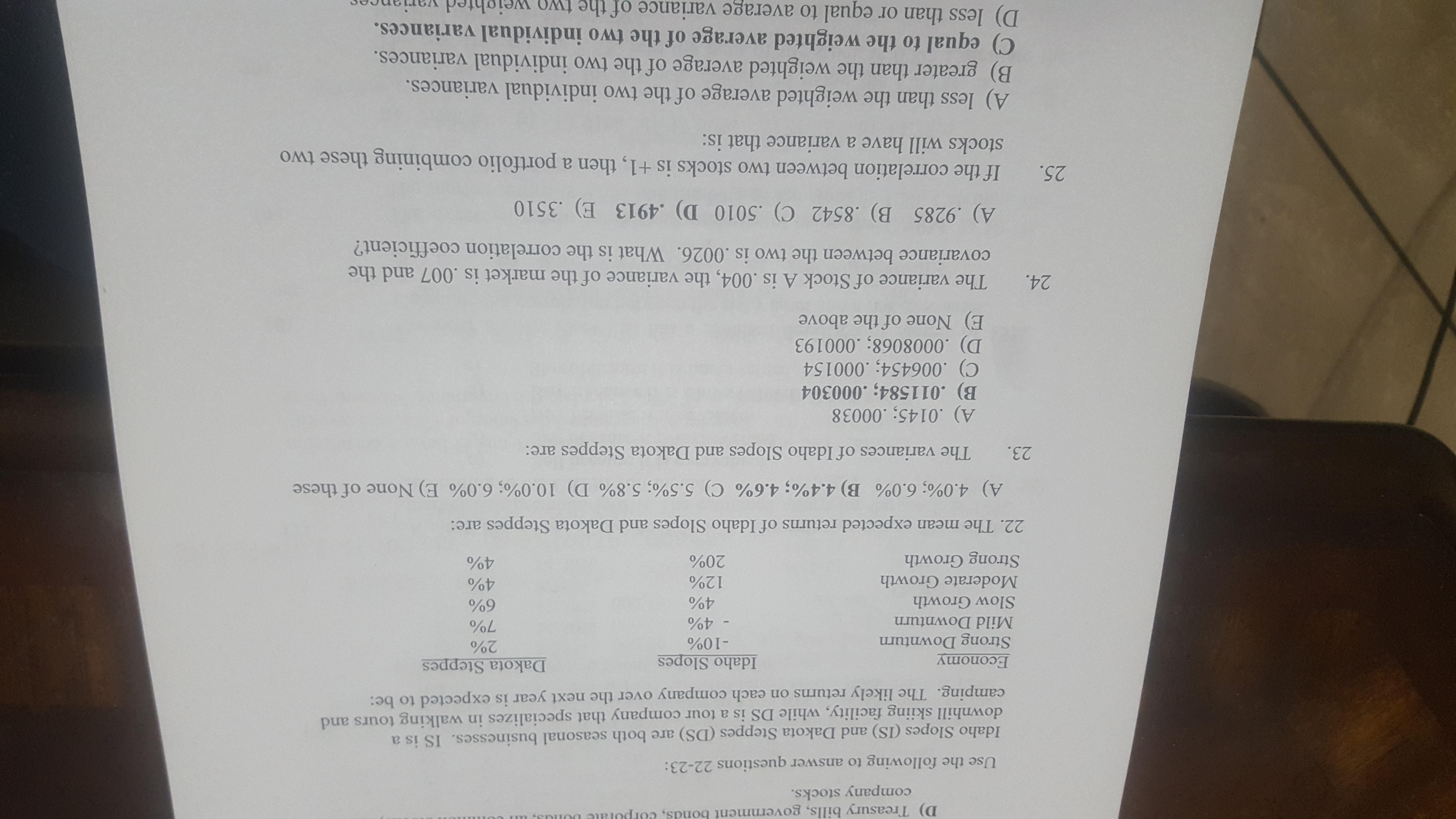# D) Treasury bills, government bonds, company stocks. Use the following to answer questions 22-23: Idaho Slopes (IS) and Dakota Steppes (DS) are both seasonal businesses. IS is a downhill skiing facility, while DS is a tour company that specializes in walking tours and camping. The likely returns on each company over the next year is expected to be: Idaho Slopes Dakota Steppes Economy Strong Downturn Mild Downturn Slow Growth %9 Moderate Growth Strong Growth 22. The mean expected returns of Idaho Slopes and Dakota Steppes are: A) 4.0%; 6.0% B) 4.4 % ; 4.6% C) 5.5 % ; 5.8% D ) 10.0%; 6.0% E ) None of these 23. The variances of Idaho Slopes and Dakota Steppes are: A) .0145; .00038 B) .011584; .000304 C) .006454; .000 1 54 D) .0008068; .000193 E) None of the above The variance of Stock A is .004, the variance of the market is .007 and the covariance between the two is .0026. What is the correlation coefficient? 24. A) .9285 B) .8542 C) .5010 D) .4913 E) .35 10 If the correlation between two stocks is +1, then a portfolio combining these two stocks will have a variance that is: 25. A) less than the weighted average of the two individual variances. B) greater than the weighted average of the two individual variances. C) equal to the weighted average of the two individual variances. D) less than or equal to average variance of the twn weighted varian

Question

I am currently working on some practice problems for my finance class and am unsure how to set up and calculate the following problems:

1) use the following information for the next two problems

you have concluded that next year the following relationships are possible:

 economic status probability rate of return weak economy .15 -5% static economy .60 5% strong economy .25 15%

a) what is your expected rate of return [E(Ri)] for next year? - I calculated this portion and got 6.00% which is the answer but part B is where I got stuck

b) compute the standard deviation of the rate of return for the one year period?

2) use the following information for the next two problems

 asset a asset b E(Ra) = 10% E (Rb) = 15% st.dev a = 8% st.dev 9.5% Wa = 0.25 Wb = 0.75

Cov AB = 0.006

a) what is the expected return of a portfolio of two risky assets if the expected return, standard deviation, covariance, and asset weight are shown as above?

b) what is the standard deviation of this portfolio?

3) what is the expected return of the three stock portfolios described below?

 common stock market value expected return ando inc 95000 12.% bee co. 32,000 8.75% cool inc. 65000 17.7%

4) the expected return for a stock, calculated using the CAPM is 10.5%. The market free return is 9.5% and the beta of the stock is 1.50. calculate the implied risk-free rate

Last three problems are attached as images (22-24)help_outlineImage TranscriptioncloseD) Treasury bills, government bonds, company stocks. Use the following to answer questions 22-23: Idaho Slopes (IS) and Dakota Steppes (DS) are both seasonal businesses. IS is a downhill skiing facility, while DS is a tour company that specializes in walking tours and camping. The likely returns on each company over the next year is expected to be: Idaho Slopes Dakota Steppes Economy Strong Downturn Mild Downturn Slow Growth %9 Moderate Growth Strong Growth 22. The mean expected returns of Idaho Slopes and Dakota Steppes are: A) 4.0%; 6.0% B) 4.4 % ; 4.6% C) 5.5 % ; 5.8% D ) 10.0%; 6.0% E ) None of these 23. The variances of Idaho Slopes and Dakota Steppes are: A) .0145; .00038 B) .011584; .000304 C) .006454; .000 1 54 D) .0008068; .000193 E) None of the above The variance of Stock A is .004, the variance of the market is .007 and the covariance between the two is .0026. What is the correlation coefficient? 24. A) .9285 B) .8542 C) .5010 D) .4913 E) .35 10 If the correlation between two stocks is +1, then a portfolio combining these two stocks will have a variance that is: 25. A) less than the weighted average of the two individual variances. B) greater than the weighted average of the two individual variances. C) equal to the weighted average of the two individual variances. D) less than or equal to average variance of the twn weighted varian fullscreen